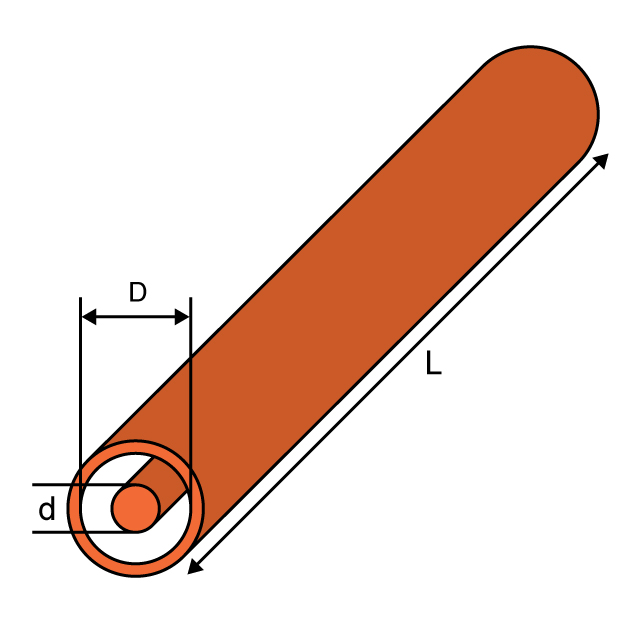# Coax Inductance Calculator

## A calculator for computing the inductance of a coaxial cable.

H

### Overview

Every form of a conductor has its own inductance, and a coaxial cable is no exception. This tool is designed to calculate the inductance of a coaxial cable if its dimensions are provided. You can select the unit of measurement for the coaxial cable's inner diameter, outer diameter, and length. The provided answer is in henries (H).

Note: The outer conductor diameter is always greater than the inner conductor diameter. Proving an inner conductor diameter that is greater than the outer conductor diameter will result in a negative inductance, which is nonsensical.### Equation

$$L_{coax} \approx \frac{\mu_{0} \mu_{r}L}{2 \pi} ln \left(\frac{D}{d}\right)$$

Where:

$$L_{coax}$$ = inductance of the coaxial cable in henries (H)

$$\mu_{0}$$ = permeability of free space = 4π×10−7

$$\mu_{r}$$ = relative permeability

$$D$$ = coaxial cable outer diameter

$$d$$ = coaxial cable inner diameter

$$L$$ = length of the coaxial cable

### Applications

When the length of a transmission line is longer than several wavelengths at the signal frequency, the two parallel conductors of the transmission line appear as a complex impedance. The wires exhibit considerable series inductance whose reactance is significant at high frequencies. In series with this inductance is the resistance of the wire, or braid making up the conductors, which includes inherent ohmic resistance plus any resistance due to skin effect. Furthermore, the parallel conductors form a distributed capacitance with the insulation, which acts as the dielectric. In addition, there is a shunt or leakage resistance or conductance (G) across the cable as the result of imperfections in the insulation between the conductors. The result is that to a high-frequency signal, the transmission line appears as a distributed low-pass filter consisting of series inductors and resistors and shunt capacitors and resistors. This is called a lumped model of a distributed line. An engineer should include in his or her design parameters the effect of the coaxial cable's inductance per unit length.